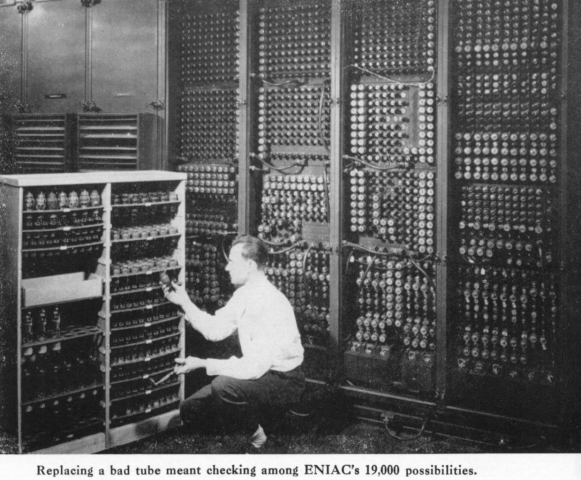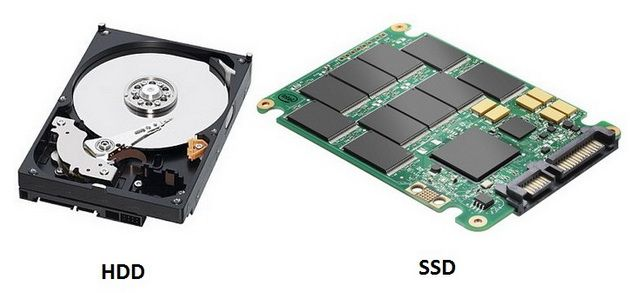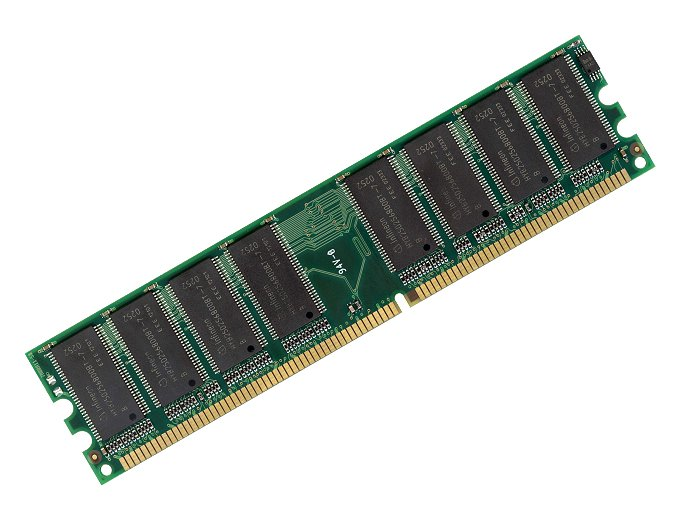Day II - Bits/Bytes  &  Memory/Storage

7. Why groups of 8?

Over sixteen million different possible colors or sounds (by using 24 binary digits per color/sound) may seem like a bit of an overkill.  You may wonder if 16 binary digits is not enough, why not just go up to, say, 17 or 18 numeric places per color/sound.  The answer is that when the ASCII code was first developed, the people involved – who were working with English letters and numbers only - figured that 256 characters would be just enough, and that more would be a waste.*  And thus, 8 binary digits (or, using the short form, 8 “bits”) became the standard unit for communicating with computers. (And actually, for a while, in the beginning the standard was just 7 bits, not 8.)  To this day, the 8 bit “byte” is the basic unit of computer communication. So multiples of eight are used if more than 256 combinations are needed since hardware/software systems are made to work with groups of 8 bits.  And, so you’ll commonly see reference to things like “24-bit color”, and “16-bit sound”.

So each individual 0 or 1, or swith on or off, etc. is a bit. And eight bits grouped together make up a byte. Or put another way: there are 8 bits in a byte.

With programming, data types are also all multiples of 8 in terms of size. In the programming language Java, the char data type is 16 bits, int and float are both 32 bits, and double is 64 bits. In fact, the number of bits a certain data type uses is the most fundamental and significant characteristic of it. How thoses bits are used - i.e., the context, whether as letters or colors or sounds - is controlled by the software/hardware of the computer, but the main distinguishing feature of an int, for example, itself, is that it resides in exactly 32 bits of memory.

pg. 115
(Analogy: the standard of a dozen, not ten eggs: If I asked you to do to the store and come back with 20 eggs, how many would you come back with?...Answer: 24 - since using the standard of 12, to get 20, we would need 2 x 12.)

* In the original computers, each bit was represented by a quite big vacuum tube.8. What about “Megabytes” and so on?

So, 8 bits make up one byte, and one byte represents one character or color or sound.  But since today’s computers deal in vast quantities of data, the units bits and bytes are limited in terms of utility.  Now-a-days we usually talk in terms of Kilobytes, Megabytes, Gigabytes, Terabytes, and Petabytes

The next step up from a byte is a KiloByte (KB).  One Kilobyte is equal to 1024 bytes.  You may wonder why 1024, and not 1000.  The reason is that in binary (two-digit) math, one thousand is not significant, whereas 1024 is, since it is 2^10.  One MegaByte (MB) is approximately one million bytes (actually 1, 048, 576 to be exact).  A Gigabyte (GB) is approximately a billion bytes, and a Terabyte is approximately a trillion bytes.
(Analogy: kilometers used for distances between cities instead of meters.)
pg. 7

9. So what is it, exactly that these units measure?

If bytes, GB and so on are the measurements, what exactly do they measure?  Well, they measure the number of groups of eight 0s and 1s used to represent different things.  This quantity is more descriptively identified as computer memory - bytes are the units used to measure computer memory.  So, for example, when we speak of a computer as having 500 Gigabytes of storage memory, we mean that it can store 500 billion groups of 8 zeros and ones.  So Memory, simply stated, is an amount of computerized data.
pg. 6-7

10. Is there a difference between storage memory and RAM memory?

Yes. We'll deal with storage memory first. Storage memory is memory which is able to be permanently stored. Often this is done with some kind of magnetic media device such as a hard drive. (The word media in this case means the type of substance on which computerized data can be stored.)  The most common type of computer magnetic media device is the hard drive, found in most desktop computers and many laptops. And there are lots of other much simpler things, such as your school lunch card, which also store data magnetically. Three other categories of storage media other than magnetic are: optical, flash, and SSD (Solid State Drives).  The three most common types of optical media are CDs, DVDs and Blu-ray discs. Flash memory is most commonly found in Flash sticks, and smaller computing devices such as phones, cameras, iPads and Macbook Airs. Solid state drives SSDs (which can be the main secondary storage of a laptop built from about 2012 on) usually use Flash memory. Sometimes the main drive of a computer/laptop will have a hybrid drive, which has both a traditional magnetic disk, as well as an SSD component.
pg. 911. What about RAM memory, then?

RAM memory is the place where data and applications are held while they are being worked on.  RAM stands for Random Access Memory.  It temporarily stores applications and data files in the form of active electric circuits; each of RAMs millions or billions of curcuits are either on or off at any given instance; it is these patterns of ons and offs that are the 1s and 0s of the binary representations of data.  Since it is precisely the memory that you are working on at one time, it is often referred to as working memory.

When you launch an application, or open a file, you are actually moving a copy of it into RAM. The reason for this is that RAM is much quicker at changing the 0s and 1s than would be the case if you worked directly from the slower hard drive. Turning on or off of electrical switches in RAM is much faster than writing or reading magnetic regions of a hard drive, which involve relatively slow physical spinning of the hard drive and rotation of the read-write head.

But, with RAM, because electricity is volatile, as soon as the electricity is turned off, all work in RAM is lost. So when changes have been made, the data is stored back to a permanent memory device such as a hard drive (i.e. it is “saved”). And so storage memory and RAM memory work hand in hand, both storing, and also changing the data.
pg. 120-121   And see the general diagram link for this unit.